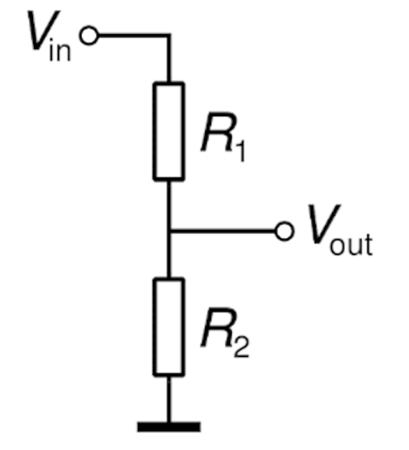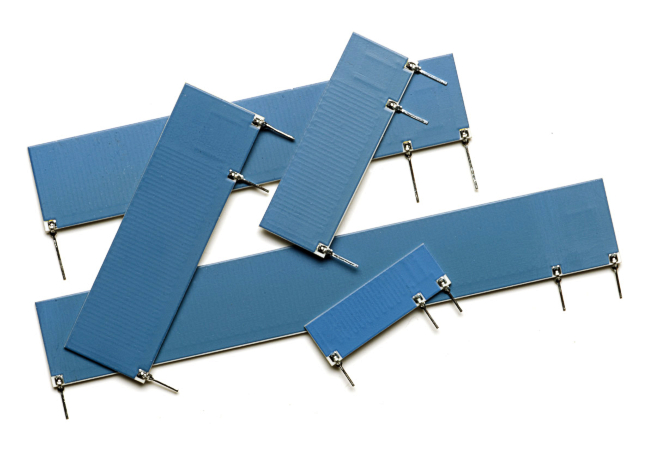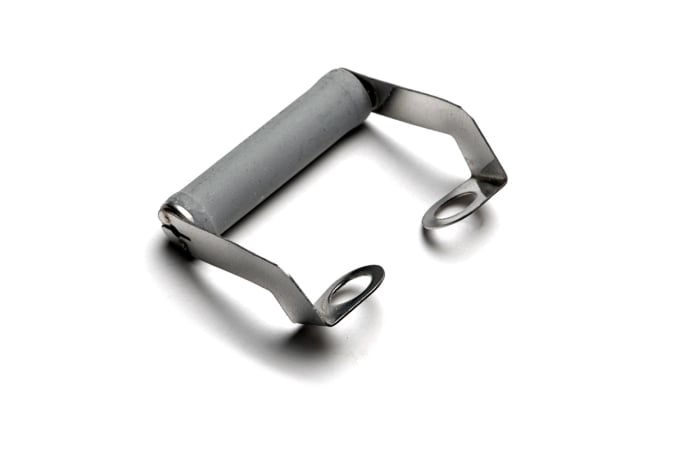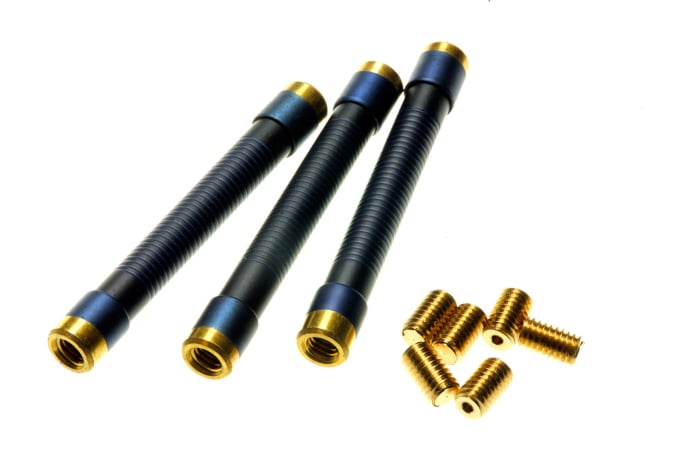Technical Article

# 10 Tips for Designing With High-voltage Resistors

March 17, 2023 by TT Electronics

## Optimized strategies prioritize the definition and testing of key components early in the design cycle. High-voltage resistors are one such component. Here are 10 tips for designing with high-voltage resistors.

Optimized strategies prioritize the definition and testing of key components early in the design cycle. “High voltage” is a term that can have several meanings, but here we are considering circuits with voltages from 1 to 100 kV.

An example from the lower end of this scale is an automatic external defibrillator, in which a capacitor is charged to up to 5 kV before delivering a potentially life-saving, precisely calibrated electrical surge to a patient.Staying with the healthcare theme but at the other end of the scale, we have medical X-rays, which are generated by accelerating electrons with around 70 kV, then abruptly stopping them with a metallic target. Carefully controlled variation in this voltage allows the system to be adjusted to capture the image of soft tissue or different bone thicknesses. These are just two examples of high-voltage applications in which resistors, usually the simplest of commodity components, are elevated in importance to provide critical protection and accurate control in demanding applications. This article presents ten tips based on support given over many years to designers needing high-voltage resistors, not just in medical but also in the industrial, transportation, and scientific sectors.

### Ten Tips

#### 1. Understanding voltage ratings

The primary voltage rating of a resistor is its limiting element voltage (LEV), sometimes called the working voltage. This is the maximum continuous voltage that may be applied across a resistor whose ohmic value is greater than or equal to the critical resistance. Below this value, the maximum voltage is restricted by the power rating $$(P_{r})\,to\,\sqrt[2\,\,\,]{}P_{r}\cdot R$$. Generally, it is DC or AC rms, but the datasheet for high voltage parts may define it as DC or AC peak. Associated with this is the overload voltage rating, which is generally 2 or 2.5 times the LEV for 2 to 5 seconds. Often, much higher peak voltages can be withstood for short durations, as indicated in the pulse performance section of a datasheet. The final rating is the isolation voltage, which is the maximum continuous voltage that may be applied between the resistor and a conductor in contact with its insulated body.

#### 2. Voltage division with discrete resistors##### Figure 1. Voltage Divider. Image used courtesy of Bodo’s Power Systems [PDF]

Voltage division requires a high-value resistor R1 in series with a low-value resistor R2, as shown in Figure 1.

The voltage ratio is given by

$\frac{V_{in}}{V_{out}}=(R_{1}+R_{2})/R_{2}=R_{1}/R_{2}+1$

It should be noted that the voltage ratio is not the same as the resistance ratio R1 / R2 but is offset by one. For example, to obtain a voltage ratio of 1000, it is necessary to define a resistance ratio of 999. For a discrete resistor design, it is preferable to select standard values, and some examples of decade voltage ratios are presented in Table 1.

##### Table 1. Decade Voltage Ratios using Standard Resistor Values
 Target Voltage Ratio R1 / R2 R1 (E12) R2 (E24 or E96) Actual Voltage Ratio Nominal Error 10 9 82K 9K1 10.01 +0.1% 100 99 470K 4K75 99.95 -0.05% 1000 999 1M0 1K0 1001 +0.1% 1000 999 6M8 6K81 999.5 -0.05% 10,000 9999 10M 1K0 10,001 +0.01%

Having selected nominal values, the next consideration is the tolerance required. The tolerance in resistance ratio is simply the sum of the individual resistance tolerances. These are not necessarily the same; often it is most economical to select a tighter tolerance on the low-voltage part. For example, high voltage R1 at 1% and low voltage R2 at 0.1% result in a resistance ratio tolerance of 1.1%. For voltage ratios exceeding 50:1, the tolerance on the voltage ratio is effectively the same as that of the resistance ratio.

#### 3. Specifying integrated voltage dividers

High-voltage dividers that integrate R1 and R2 into one three-terminal component are available, illustrated by TT Electronics’ HVD series (Figure 2). There are a number of precision advantages to this approach. For example, the target voltage ratio may be defined precisely without the constraint of choosing standard values.##### Figure 2. Integrated Voltage Dividers. Image used courtesy of Bodo’s Power Systems [PDF]

The values specified for integrated dividers are normally the low-value R2 and the total value R1 + R2. Also, the tolerance on the voltage ratio can be controlled directly by the trimming process and so can be made considerably tighter than the absolute tolerances on the resistor values. For example, R1 and R2 can be defined with 2% absolute tolerances, but the voltage ratio may be adjusted to a 0.5% tolerance. A similar advantage can apply in relation to the temperature coefficient of resistance (TCR), with the tracking TCR, which determines the temperature stability of the voltage ratio, being potentially lower than the absolute TCR of the resistor elements. Further, it is possible to design dividers that extend this element of matching to the areas of life drift and voltage coefficient of resistance (VCR), although this will typically call for a customized design.

#### 4. Evaluating TCR and VCR errors in dividers

Provided the R1 value is sufficiently high, and the voltage sufficiently low, there will be a low level of self-heating in the divider. If this is the case, it is relatively easy to measure the TCR and VCR effects separately. TCR effects are calculated using a temperature chamber, and the resulting figure of merit is defined as Temperature Coefficient of Voltage Ratio $$=\frac{1E+6\cdot\frac{VRht-VRlt}{VRlt}}{HT-LT}$$ in ppm/°C where VRht and VRlt are the voltage ratios at high and low temperatures, and HT and LT are the high and low temperatures.

The corresponding figure of merit for VCR effects is similarly defined as Voltage Coefficient of Voltage Ratio $$=\frac{1E+6\cdot\frac{VRhv-VRlv}{VRlv}}{HV-LV}$$ in ppm/°V where VRhv and VRlv are the voltage ratios at high and low voltages, and HV and LV are the high and low voltages.

If self-heating is not negligible, then in the TCR test, the chamber temperature should be adjusted to give the correct HT figure, and time should be allotted for the temperature to stabilize. The VCR test should be short in duration to minimize temperature rise. Alternatively, one can use a temperature chamber to measure low voltages at higher temperatures and vice versa, thereby canceling out temperature-related resistance changes.

#### 5. Calculating the value of a bleed resistor

Bleed resistors are used to discharge capacitors to safe voltage levels after power is removed. A bleed resistor may be either switched across the capacitor for rapid discharge without quiescent dissipation or permanently connected for high reliability and low cost. In the latter case, there is a trade-off between the time to reach safe discharge and the quiescent power loss. Selecting a maximum suitable ohmic value is achieved from an exponential discharge calculation:

$R_{max}=-TD/C\cdot Ln(V_{t}/V_{o})$

where Td is discharge time, C is capacitance value assuming maximum positive tolerance, Vt is the safety threshold voltage, and Vo is the initial voltage. The highest standard value, which, allowing for tolerance, lies below Rmax should be used.

For a selected value R, the initial power is given by Po = Vo 2/R . For a switched bleeder, this is the peak power. For a permanently connected bleeder, it is the continuous dissipation, and the resistor chosen must be rated accordingly.

#### 6. Selecting the right balancing resistor

All aluminum electrolytic capacitors exhibit a leakage current when a DC voltage is connected across them. This may be modeled by a leakage resistance connected in parallel with the capacitor. This resistance is non-linear, that is, its value is a function of the applied voltage. In this case, the value is poorly defined, having a large degree of variation from one capacitor to another. When building a capacitive reservoir for a high-voltage DC bus, it may be necessary to use a series combination of two capacitors, each rated at half the bus voltage. If the capacitors are identical, the bus voltage will be shared equally between them. However, in practice, the leakage resistances will differ, leading to uneven sharing and possible voltage overload on the capacitor with the higher leakage resistance.##### Figure 3. TT Electronics’ WPYP series is designed for direct mounting on capacitors. Image used courtesy of Bodo’s Power Systems [PDF]

The solution is to use balancing resistors, such as that shown in Figure 3, in parallel with each capacitor. These are high-value resistors rated at the appropriate voltage and matched in value to within a few percent. The value needs to be as high as possible to minimize power dissipation but is generally chosen so that it is no more than 10% of the lowest value of leakage resistance at the rated voltage of the capacitor. By this means, the effect of the unbalanced internal capacitor leakage resistance is swamped by that of the balancing resistors, and the voltages are approximately equalized.

#### 7. Withstanding high voltage surges

It is sometimes the case that designers looking at high-voltage resistors are doing so because their circuits must withstand high-voltage transients. If the continuous voltage stress does not call for a high-voltage rating, it may well be the case that a low-voltage but surge-tolerant part is the best solution. For example, TT Electronics’ 5W wire-wound high surge resistor, WH5S, does not have a high-voltage rating but can withstand a 1.2/50µs up to 10kV peak, whilst the surge tolerant 2512 chip resistor, HDSC2512, has an LEV of 500V but can withstand a peak voltage of up to 7kV.

#### 8. Designing to meet safety standards

When designing equipment to meet the requirements of electrical safety standards such as IEC 60664, it is necessary at an early stage to consider the relevant creepage and clearance requirements. These will not only affect PCB layout design but also, in some cases, component selection. Where a resistor connects to a high-voltage level, it is important to check the distance between its terminations and, in the case of heatsink-mounted parts, between the resistor and a metallic thermal interface. This is defined in two ways. Firstly, the creepage is the shortest distance across an insulating surface. This reduces the likelihood of humid and contaminating conditions enabling surface scintillations with energy high enough to entail tracking. Secondly, the clearance is the shortest distance in the air. This addresses the risk of flashover. These two dimensions, if not apparent from datasheets, should be available from the manufacturer.

Another piece of information that may be needed is the material forming the insulating surfaces, as this determines the comparative tracking index (CTI), which classifies an organic material’s propensity to support processes leading to track. If a resistor bridges the isolation barrier in a design, for example, to provide a galvanic connection to prevent excessive electrostatic charge build-up, the IEC 60065 safety standard requires resistors to withstand a specified high-voltage surge test. As this is becoming a legacy standard, ongoing certification of resistors is no longer relevant. Still, designers following the hazard-based safety engineering approach of IEC 62368-1 will be helped in knowing that there are still products that meet the requirements of IEC 60065.#### 9. Optimising the PCB layout

PCB layout is crucial to maintaining the safety of a high-voltage design, and this is most obvious where high-voltage resistors are miniaturized and in surface mount device (SMD) form. A good example is TT Electronics’ HVC series which includes a 2512-size chip resistor with a 3kV rating. Tracks or vias beneath or very close to the component should be avoided, as should any features likely to trap or encourage ionic contamination during manufacture or use. One special measure which may be used to increase creepage distance and avoid trapped contamination is the cutting of a slot in the PCB beneath the component.

#### 10. Designing for potted and oil-filled assemblies

Two limiting factors in high voltage designs can be the tendency of contaminated organic surfaces to support tracking and the risk of electrical discharge in the air, particularly around small radius surfaces. Both of these constraints can be tackled by potting or immersion in mineral oil, which prevents the ingress of contamination and replaces air with a substance of higher dielectric strength. This, in turn, reduces creepage and clearance constraints allowing an assembly to be reduced in size. When choosing resistors for such an assembly, it is essential to select parts that are insulated in a manner that avoids the risk of outgassing. Any air incorporated with the component can form a void in which partial discharges can occur, leading to the long-term degradation of insulating materials. This rules out the use of parts with insulation sleeving or with rough or porous coating finishes. An epoxy coating, either printed or powder dipped, is often ideal, and a manufacturer can advise on suitability.

### Conclusion

In many cases, resistors can be regarded as the simplest of components in a circuit and need no special attention from designers beyond selecting an appropriate ohmic value and power rating. However, high-voltage circuits often call for a specialist component from a manufacturer who can provide experience and expertise. The designer is well advised to prioritize these as critical components for definition and testing at an early stage in the project and to check whether a custom or semi-custom approach can add significant value.